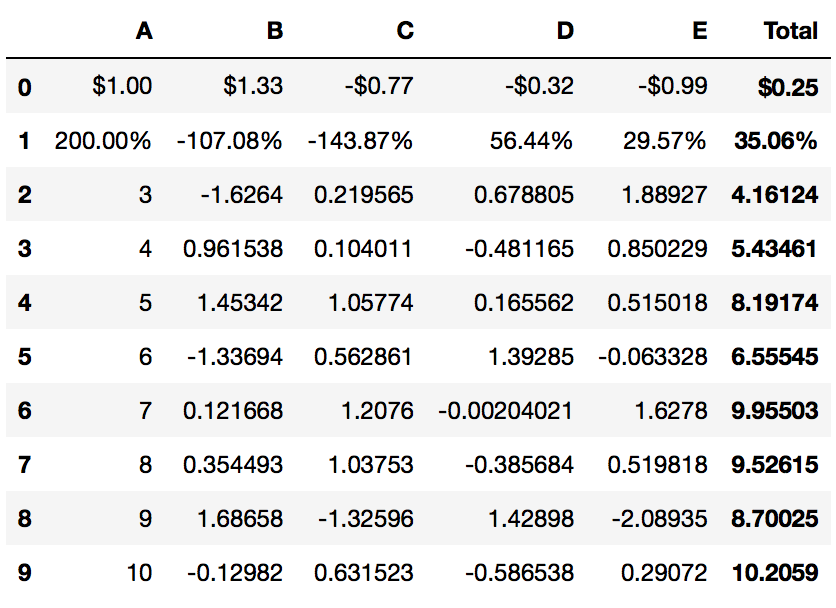# Quick Start¶

PrettyPandas supports many built in summary functions, as well as providing the ability to create your own summaries. Summary functions can be applied over a DataFrame’s rows or columns, or both.

The builtin summary methods are:

If you wanted to add a grand total to the bottom of your table the code is simple:

```PrettyPandas(df).total()
```Or additionally if you want to use Pandas fluent API:

```df.pipe(PrettyPandas).total()
```

PrettyPandas follows a fluent API so you can chain multiple summaries easily:

```df.pipe(PrettyPandas).total().average()
```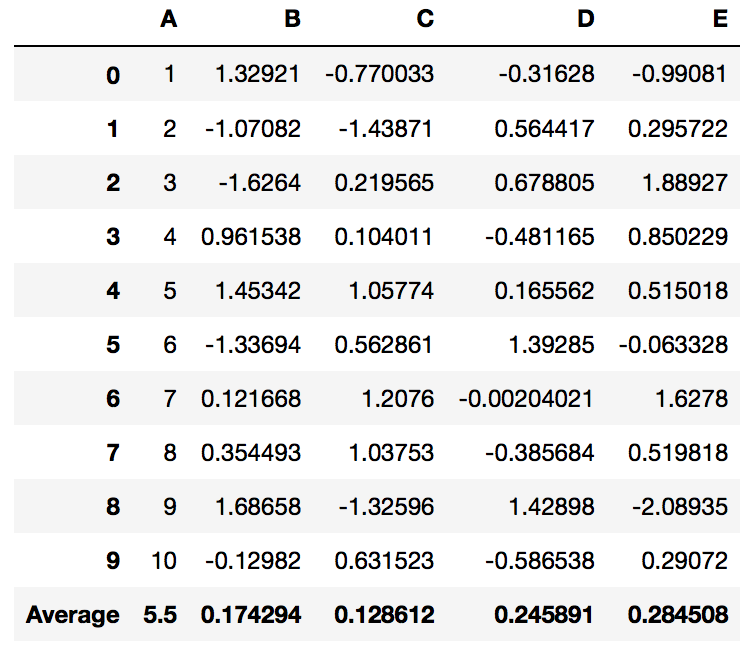The `axis` parameter specifies which `numpy` style axis to apply a summary on — 0 for columns, 1 for rows, and `None` for both.

```PrettyPandas(df).total(axis=1)
```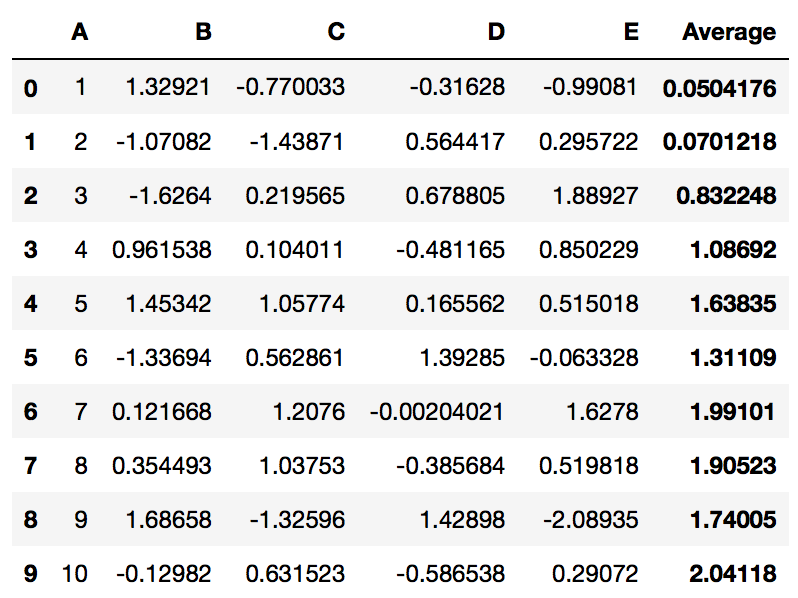You can even mix and match summaries applied to different axis.

### Creating a Custom Summary¶

The `summary` method creates a custom summary from a function which takes an array-like structure as a list.

```def count_greater_than_zero(column):
return (column > 0).sum()

PrettyPandas(df).summary(count_greater_than_zero, title="> 0")
```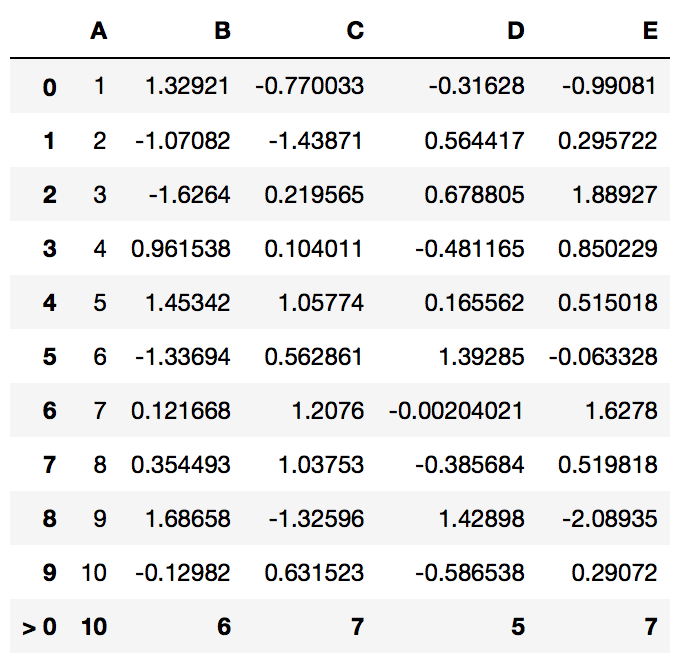## Converting Back to Pandas DataFrame¶

### `.to_frame()`¶

After adding summary rows or columns you can get a DataFrame with your changes applied by calling the `._to_frame`.

For example the following code would add a total to your DataFrame and return it back to a Pandas native DataFrame.

```(
df
.pipe(PrettyPandas)
.total(axis=1)
.to_frame()
)
```

### `.style`¶

The `.style` property allows you to drop right into the Pandas Style API. This code would allow you to compute a summary, format the table using percentages, and apply a backgrouned gradient to a table:

```(
df.pipe(PrettyPandas)
.as_percent(precision=0)
.median()
.style
)
```

## Formatting Numbers¶

Most reports use at least some units of measurement. PrettyPandas currently supports percentages, money, and a more general unit method.

The `as_unit` method takes a positional `unit` argument which indicates the string representing the unit to be used and a `location` argument to specify whether the unit should be a prefix or suffix to the value.

The `as_currency` and `as_percent` methods are localized to use whatever units your Python distribution thinks are best for you. If you aren’t getting the correct units use the `set_locale` method to specify your locale.

If you need to use a different currency, just pass it to `currency='...'` to change it.

The `as_money` method takes optional `currency` and `location` arguments which work just like the `as_unit` method. By default the currency is in dollars.

Note

Python 2 doesn’t support unicode literals by default. You can use unicode literals (e.g. `u'€'`) or import the unicode literal behaviour from Python 3:

```from __future__ import unicode_literals
```

### Formatting Columns¶

By default the formatting methods apply to the entire dataframe. When you need to format just a few columns you can use the subset argument to specify a single column, or multiple columns.

```PrettyPandas(df).as_percent(subset='A')  # Format just column A
``````PrettyPandas(df).as_percent(subset=['A', 'B'])  # Format columns A and B
```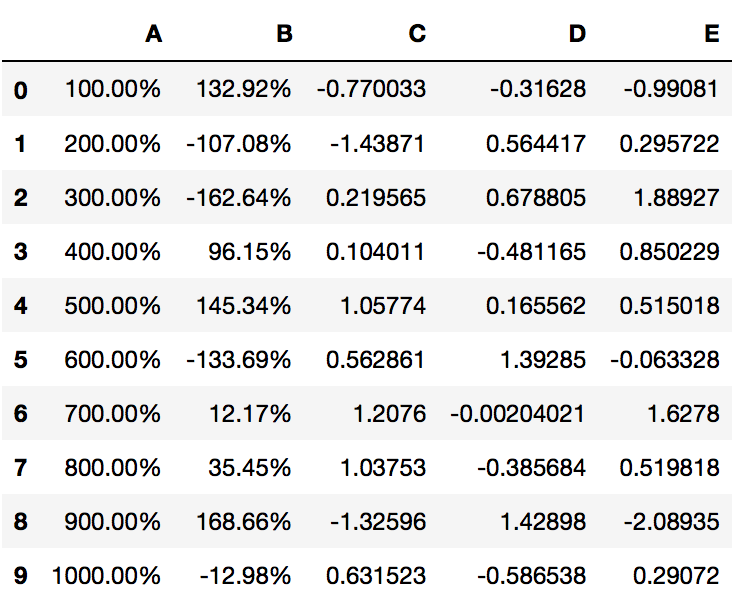### Formatting Rows and Complex Formatting¶

Formatting rows is more complicated than formatting columns. The subset argument needs to take in a pandas.Index to specify the row.

```# Format the row with row-index 3
PrettyPandas(df).as_percent(subset=pd.IndexSlice[3,:], precision=2)
```For multi-index dataframes subsetting is more complicated. You will need to use multiple `pandas.IndexSlice` objects to get the correct rows.

The following example shows how to select rows in a multi-index:

```first_row_idx = pd.IndexSlice[0, :]
second_row_idx = pd.IndexSlice[1, :]

(
df.pipe(PrettyPandas)
.as_currency(subset=first_row_idx)
.as_percent(subset=second_row_idx)
.total(axis=1)
)
```